# T1 is hereditary

This article gives the statement, and possibly proof, of a topological space property (i.e., T1 space) satisfying a topological space metaproperty (i.e., subspace-hereditary property of topological spaces)
View all topological space metaproperty satisfactions | View all topological space metaproperty dissatisfactions
Get more facts about T1 space |Get facts that use property satisfaction of T1 space | Get facts that use property satisfaction of T1 space|Get more facts about subspace-hereditary property of topological spaces

## Statement

Any subspace of a T1 space, endowed with the subspace topology, is again a$T_1$-space.

## Definitions used

### T1 space

Further information: T1 space

A topological space$X$ is termed$T_1$ if it satisfies the following equivalent conditions:

1. For any two distinct points$x,y \in X$, there exists an open subset$U$ of$X$ such that$x \in U, y \notin U$
2. For every point$x \in X$, the singleton subset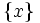$\{ x \}$ is closed in$X$
3. For every point$x \in X$, the intersection of all open subsets of$X$ containing$x$, is$\{ x \}$

### Subspace topology

Further information: Subspace topology

The subspace topology on a subset$A$ of$X$ is defined in the following equivalent ways:

1. A subset$U$ of$A$ is open in$A$ iff there exists an open subset$V$ of$X$ such that$V \cap A = U$.
2. A subset$C$ of$A$ is closed in$A$ iff there exists a closed subset$D$ of$X$ such that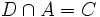$D \cap A = C$.

## Proof

### Proof in terms of two-point definition of T1

Given: A$T_1$-space$X$, a subset$A$

To prove:$A$ is a$T_1$-space when endowed with the subspace topology

Proof: We need to show that if$x \ne y$ are both points of$A$, then there exists an open subset of$A$ containing$x$ and not containing$y$.

Since$x,y$ are distinct points of$A$, they are also distinct points of$X$. Since$X$ is a$T_1$-space, there exists an open subset$V$ of$X$ such that$x \in V$ and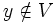$y \notin V$. Now consider the set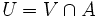$U = V \cap A$. Then, by definition of subspace topology,$U$ is open in$A$. Further, since$x \in A$ and$x \in V$, we have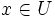$x \in U$. Since$y \notin V$, we have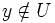$y \notin U$. Thus,$U$ is an open subset of$X$ containing$x$ and not containing$y$.